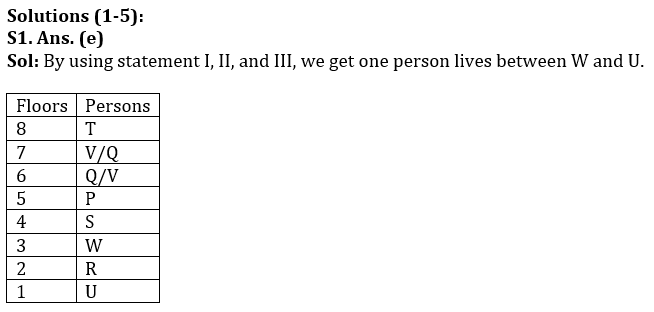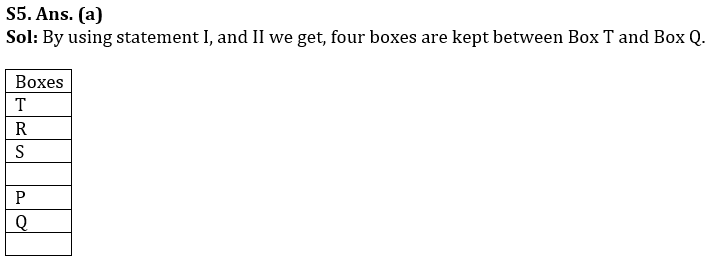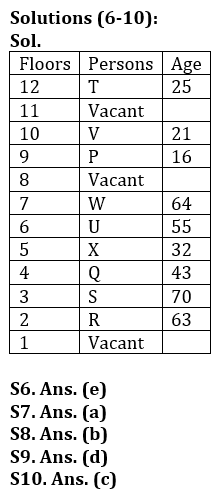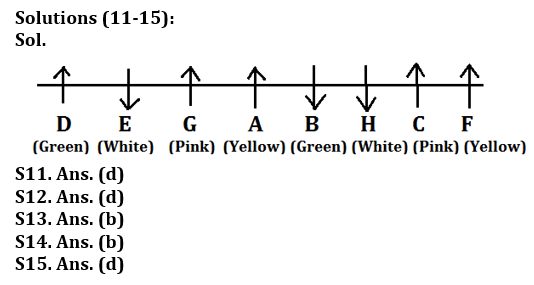Latest Banking jobs   »

# Reasoning Ability Quiz For LIC AAO Mains 2023-2nd March

Directions (1-5): Each of the questions below consists of a question and three statements numbered I, II and III given below it. You have to decide whether the data provided in the statements are sufficient to answer the question. Read both the statements and given answer.

Q1. Eight persons P, Q, R, S, T, U, V and W, are living in a building of eight floors such that the ground floor is numbered as 1 and the topmost floor is numbered as 8 but not necessarily in the same order. How many persons live between W and U?
(I) Only two persons live between R and P. P lives on an odd number floor above R.
(II) Only three persons live between T and S who lives on the 4th floor.
(III) Only three persons live between P and U. V and Q does not live below S.

(a) If the data in statement I and II are sufficient to answer the question.
(b) If the data in statement II and III are sufficient to answer the question.
(c) If the data in statement I and III are sufficient to answer the question.
(d) If the data in all the statement I, II and III together are not sufficient to answer the question.
(e) If all the statements I, II and III are necessary to answer the question.

Q2. In which day of the week does Y take a leave, assuming all seven days are working days?
(I) Y’s brother correctly remembers that Y went to office on Wednesday.
(II) Y correctly remembers that he took a leave before Saturday but not on Friday.
(III) Y’s father correctly remembers that Y took a leave after Monday.

(a) If the data in statement I and II are sufficient to answer the question.
(b) If the data in statement II and III are sufficient to answer the question.
(c) If the data in statement I and III are sufficient to answer the question.
(d) If the data in all the statement I, II and III together are not sufficient to answer the question.
(e) If all the statements I, II and III are necessary to answer the question.

Q3. What will be the code for ‘cunning fellow’?
(I) ‘fellow are demanded’ is coded as ‘mr pa or’
(II) ‘cunning fellow are’ is coded as ‘mr pa rk’
(III) ‘cunning is dangerous’ is coded as ‘rk lm cr’

(a) If the data in statement I and II are sufficient to answer the question.
(b) If the data in statement II and III are sufficient to answer the question.
(c) If the data in statement I and III are sufficient to answer the question.
(d) If the data in all the statement I, II and III together are not sufficient to answer the question.
(e) If all the statements I, II and III are necessary to answer the question.

Q4. Seven persons P, Q, R, S, T, U, and V are sitting in a straight row. Who sits on the extreme right end if all of them are facing north direction?
(I) Three persons sit between S and R, and neither of them sits at the extreme ends.
(II) Only one person sits between P and U who sits immediate left of R.
(III) Only two person sits between Q and V who does not sit near to S.

(a) If the data in statement I and II are sufficient to answer the question.
(b) If the data in statement II and III are sufficient to answer the question.
(c) If the data in statement I and III are sufficient to answer the question.
(d) If the data in all the statement I, II and III together are not sufficient to answer the question.
(e) If all the statements I, II and III are necessary to answer the question.

Q5. Seven boxes P, Q, R, S, T, U, and W are kept one above another but not necessarily in the same order. How many boxes are kept between Box Q and Box T?
(I) Only one box is kept above box R and three boxes are kept between box Q and box R. Box T is kept above box P but not immediately above.
(II) The number of boxes kept below box S is same as above box P which is kept just above box Q.
(III) Box W is kept below box U but not immediate below.

(a) If the data in statement I and II are sufficient to answer the question.
(b) If the data in statement II and III are sufficient to answer the question.
(c) If the data in statement I and III are sufficient to answer the question.
(d) If the data in all the statement I, II and III together are not sufficient to answer the question.
(e) If all the statements I, II and III are necessary to answer the question.

Directions (6-10): Read the following information carefully and answer the following questions.
Nine persons P, Q, R, S, T, U, V, W, and X lives in a building of twelve floors such that the ground floor is numbered as 1 and the topmost floors is numbered as 12 but not necessarily in the same order. They all different age viz. 21 years, 16 years, 25 years, 32 years, 63 years, 64 years, 70 years, 55 years, and 43 years but not necessarily in the same order.
Note: The person having odd number of age lives on even number floor and vice-versa.
W’s age is a perfect square, but he does not live on an even number floor. X is older than T, who is older than V.
S does not live on the 10th floor. The number of floors above P is same as the number of floors below Q. The one whose age is half of the person living on floor number 5 lives on the 9th floor. Three persons live between the one whose age is 55 years and the one whose age is 63 years and both of them lives below floor number 8. Only three persons live between P whose age is 16 years and the one whose age is 43 years. The one whose age is 43 years lives below P. The number of floors between P and Q is same as the number of floors between R who lives on the 2nd floor and the one whose age is a perfect square. There is only one floor between the one whose age is 25 years and the one whose age is 21 years and both of them lives above floor number 7. The one whose age is the highest does not live above Q. P lives immediate above one of the vacant floors. There are two floors between S and U who age is a multiple of 11. T lives immediately above one of the vacant floors.

Q6. The number of persons living between P and X is same as in person living between ____ and ____?
(a) T and P
(b) P and U
(c) R and X
(d) V and U
(e) Both (c) and (d)

Q7. How many persons live below X?
(a) Three
(b) Four
(c) One
(d) Two
(e) Cannot be determined

Q8. How many persons are living between X and T?
(a) Three
(b) Four
(c) Six
(d) Seven
(e) None of these

Q9. Four of the following five are alike in a certain way based on a group, who among the following does not belong to that group?
(a) T
(b) V
(c) R
(d) X
(e) U

Q10. What is the age of the person who lives immediately above V?
(a) 21 years
(b) 25 years
(c) The floor is vacant
(d) 63 years
(e) None of these

Direction (11-15): Study the following information and answer the given questions:
Eight persons are sitting in a row either facing north or south direction. They like different colours – Green, Yellow, White and Pink such that two persons like same colours. No two persons who like same colours sit together. Not More than two persons facing same direction sit together.
Those who like Pink faces north. Three persons sit between A and F. F like yellow and sit at one of the ends. H, who likes White sits second to the right of A and faces opposite direction of A. The one who likes Pink sits third to the right of H. The one who likes green sits at one of the extreme ends and faces north. Neither E nor G sits at any end. C likes Pink and is not a neighbor of A. A neither likes Green nor White. Those who like yellow faces same direction. E, who sits to the immediate left of G does not like Pink. B neither sits to the left of D nor faces north.

Q11. Who among the following sits immediate right of A?
(a) C
(b) E
(c) G
(d) B
(e) None of these

Q12. What is the position of C with respect to G?
(a) Immediate right
(b) Third to the left
(c) Second to the left
(d) Fourth to the right
(e) None of these

Q13. Which of the following is true about E?
(a) E sits immediate right of the one who likes pink
(b) E likes white
(c) B sits third to the right of E
(d) E sits third from one of the ends
(e) Both (b) and (c)

Q14. Which of the following group of persons likes Yellow colour?
(a) D-A
(b) F-A
(c) H-F
(d)B-G
(e) C-E

Q15. Who among the following person sits second to the left of F?
(a) B
(b) G
(c) A
(d) H
(e) None of these

SolutionsS2. Ans. (d)
Sol: By using all the statement we get that Y took a leave on either Tuesday or Thursday.
S3. Ans. (d)
Sol: By using all the statement we get the code for ‘cunning fellow’ as either ‘rk mr’ or ‘rk, pa’.
S4. Ans. (d)
Sol: By using all the statement we cannot find who among the following sits at the extreme right end of the row.## FAQs

### When will the LIC AAO Mains exam be held?

LIC AAO Mains exam will be held on 18 March

#### Congratulations!Union Budget 2023-24: Free PDF